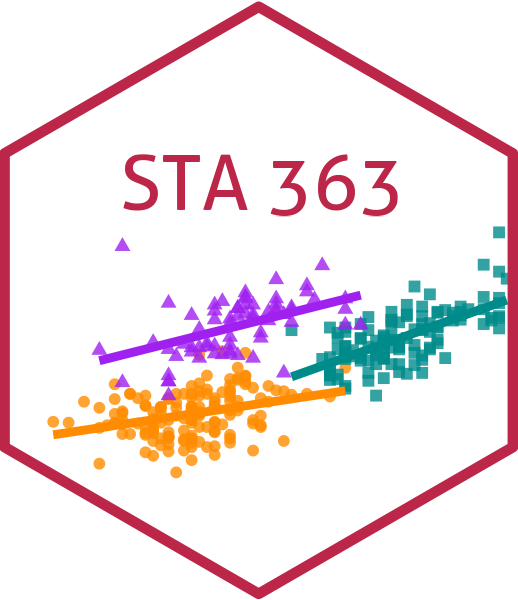# Exploratory Data Analysis

Lucy D’Agostino McGowan

## Learning objectives

• Identify data types and matching appropriate visualization techniques
• Explain the utility of exploratory data analysis
• Conduct exploratory data analysis on a new dataset

## Why EDA?

• Check whether everything is as expected in your data
• Are there as many rows as you expected in the data set?
• Is any data missing?
• Are there data entry errors?
• Are there outliers?

## Why EDA?

• Check whether everything is as expected in your data
• Check whether the assumptions of your modeling choice are met
• Do the data types match the analysis method?
• If doing simple linear regression is the relationship linear?
• If doing multiple linear regression, is the functional form modeled correctly?
• Are any points having a strong influence on the model results?

Some data is already loaded when you load certain packages in R, to access these, you just need to use the data() function like this:

library(datasauRus)
data(datasaurus_dozen)

Other times you’ll have data in a file, like a .csv or Excel file. You can use read_* functions that load when you load the tidyverse package to read these in. For example, to read a .csv file in, you could run:

movie_data <- read_csv("movie_data.csv")

Note, movie_data.csv would need to be saved in your RStudio project folder for this code to run. We will practice this in a few weeks.

## glimpse at your data

glimpse(datasaurus_dozen)
Rows: 1,846
Columns: 3
$dataset <chr> "dino", "dino", "dino", "dino", "dino", "d…$ x       <dbl> 55.3846, 51.5385, 46.1538, 42.8205, 40.769…
$y <dbl> 97.1795, 96.0256, 94.4872, 91.4103, 88.333… How many rows are in this dataset? How many columns? 00:30 ## glimpse at your data library(palmerpenguins) glimpse(penguins) Rows: 344 Columns: 8$ species           <fct> Adelie, Adelie, Adelie, Adelie, …
$island <fct> Torgersen, Torgersen, Torgersen,…$ bill_length_mm    <dbl> 39.1, 39.5, 40.3, NA, 36.7, 39.3…
$bill_depth_mm <dbl> 18.7, 17.4, 18.0, NA, 19.3, 20.6…$ flipper_length_mm <int> 181, 186, 195, NA, 193, 190, 181…
$body_mass_g <int> 3750, 3800, 3250, NA, 3450, 3650…$ sex               <fct> male, female, female, NA, female…
\$ year              <int> 2007, 2007, 2007, 2007, 2007, 20…

What type of variable is species? How many numeric variables are there?

00:30

# Visualizing data

## What are we looking for?

• The “shape” of the data
• Patterns
• Outliers (strange points / data errors)

## Data

Let’s grab one of the datasaurus_dozen datasets.

x_data <- datasaurus_dozen |>
filter(dataset == "x_shape")

What does filter do? Why ==?

00:30

# One continuous variable

## Histogram

The geom_* in ggplot2 describe the type of plot you want to create. What do you think would create a histogram?

00:30

## Histogram

ggplot(x_data, aes(x = x)) +
geom_histogram()
stat_bin() using bins = 30. Pick better value with binwidth.

What does this warning mean? How do you think we can get rid of it?

## Histogram

What does this plot tell us about the shape of this data?

00:30

## Density plot

What geom_ do you think would create a density plot?

00:30

geom_density

## Density plots

ggplot(x_data, aes(x = x)) +
geom_density()

## Boxplot

What geom_ do you think would create a boxplot?

00:30

## Boxplot

Does this give us as much information as the histogram?

00:30

## Boxplot

ggplot(x_data, aes(x = x, y = 1)) +
geom_boxplot() +
geom_jitter()

## Boxplot

ggplot(x_data, aes(x = x, y = 1)) +
geom_boxplot() +
geom_jitter()

# Relationship between two continuous variables

## Scatterplot

ggplot(x_data, aes(x = x, y = y)) +
geom_point()

## Hex plot

ggplot(x_data, aes(x = x, y = y)) +
geom_hex()

# One categorical variable

## Barplot

ggplot(datasaurus_dozen, aes(x = dataset)) +
geom_bar()

What does this plot tell us?

00:30

## Barplot

ggplot(datasaurus_dozen, aes(x = dataset)) +
geom_bar() +
coord_flip()

# Relationship between continuous and categorical variables

## Histogram

ggplot(datasaurus_dozen, aes(x = x, fill = dataset)) +
geom_histogram(bins = 30, alpha = 0.5)

## Histogram

ggplot(datasaurus_dozen, aes(x = x)) +
geom_histogram(bins = 30) +
facet_wrap(~dataset)

## Histogram

smaller_data <- datasaurus_dozen %>%
filter(dataset %in% c("slant_down", "x_shape"))

What does %in% do?

00:30

## Histogram

ggplot(smaller_data, aes(x = x, fill = dataset)) +
geom_histogram(bins = 30, alpha = 0.5)

## Ridge plots

library(ggridges)
ggplot(datasaurus_dozen, aes(x = x, y = dataset, fill = dataset)) +
geom_density_ridges(alpha = 0.4, bandwidth = 2)

## Boxplot

ggplot(datasaurus_dozen, aes(x = x, y = dataset)) +
geom_boxplot()

What is missing?

00:30

## Boxplot

ggplot(datasaurus_dozen, aes(x = x, y = dataset)) +
geom_boxplot() +
geom_jitter()

How can we make this more legible?

00:30

## Boxplot

ggplot(datasaurus_dozen, aes(x = x, y = dataset, color = dataset)) +
geom_boxplot() +
geom_jitter(alpha = 0.5)

## How will we use this?

• Plot every outcome variable before performing an analysis
• Be sure to include labels and titles on all plots for full points
• Plot important features
• Be sure to note any missing data patterns

## Application Exercise

1. Open the Welcome Penguins folder from the previous application exercise

2. Create a boxplot examining the relationship between the body mass of a penguin and their species.

3. Add jittered points to this plot

4. Add labels and a title to this plot

08:00